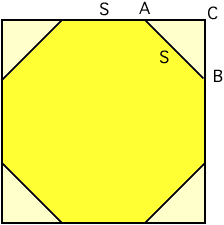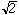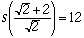If I have an octagon that is 12 feet across (side to opposite side) how can I find the length of the sides? THank you Hi, I assume you have a regular octagon so you can think of it as a 12 ft by 12 ft square with the corners cut off. Suppose that each side has length S.In the diagram the corner triangle, ABC, has |CA| = |BC| and thus using the theorem of Pythagoras |CA|2 + |BC|2 = S2 2 |CA|2 = S2 |CA| = S/Since the octagon is 12 feet across, S + 2(S/) = 12S = 4.97 feet Cheers, Harley Go to Math Central